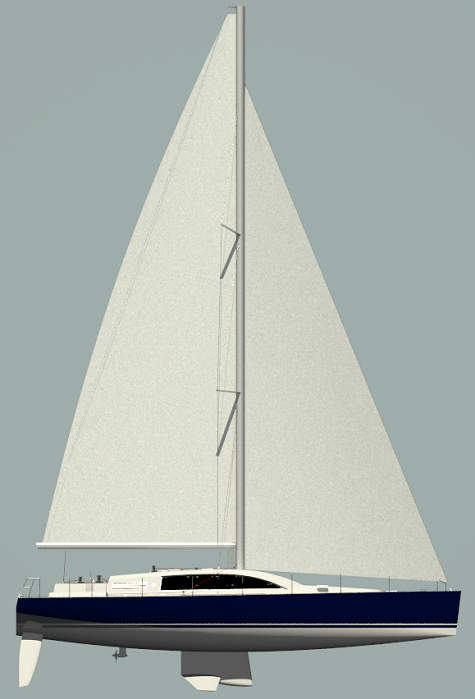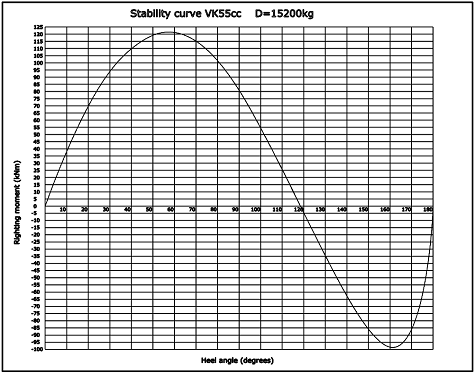SailsVK55cc Technical data Length overall = 16,8m Length waterline = 16,5m Beam overall = 4,8m Beam waterline = 3,6m Depth midsection = 4,3m Free board midsection = 1,67m Draft = 2,13m Displacement = 15200kg Ballast = 4700kg Engine VH4.80-59kW(80.3hp) Mainsail = 84m2 Genoa = 80m2 Spinnaker = 260m2 I = 18.8m J = 7.6m P = 20m E = 7.2m RM30 = 93,8kNm Water = 340l Calorifier = 55l Fuel = 220l Gray&black = 120l Boat design category CE: A+ Certification: Ocean plus Design: VK Yacht

Stability curve